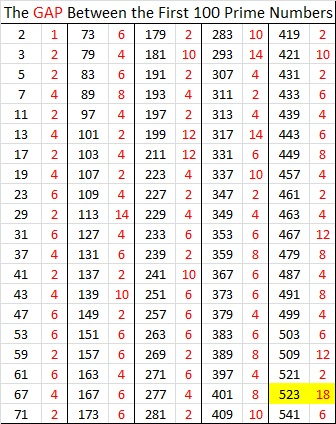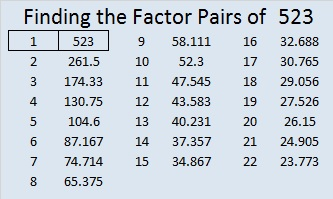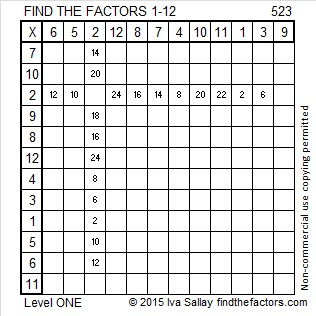523 and Level 1

523 = 61 + 67 + 71 + 73 + 79 + 83 + 89 which are all the prime numbers between 60 and 96.

521 and 523 are twin primes.

523 is the 99th prime number. It will take longer than ever before for another prime number to be featured on this blog.

The prime gap is defined as the difference between two consecutive prime numbers. The gap between this prime number and the next one is greater than between any two previous prime numbers.Here is today’s puzzle:Print the puzzles or type the solution on this excel file: 12 Factors 2015-06-15

—————————————————————————————————

• 523 is a prime number.
• Prime factorization: 523 is prime.
• The exponent of prime number 523 is 1. Adding 1 to that exponent we get (1 + 1) = 2. Therefore 523 has exactly 2 factors.
• Factors of 523: 1, 523
• Factor pairs: 523 = 1 x 523
• 523 has no square factors that allow its square root to be simplified. √523 ≈ 22.869193How do we know that 523 is a prime number? If 523 were not a prime number, then it would be divisible by at least one prime number less than or equal to √523 ≈ 22.9. Since 523 cannot be divided evenly by 2, 3, 5, 7, 11, 13, 17, or 19, we know that 523 is a prime number.

—————————————————————————————————